# Termination criteria

This section describes the stopping tests used by Knitro to declare (local) optimality, and the corresponding user options that can be used to enforce more or less stringent tolerances in these tests.

## Continuous problems

The first-order conditions for identifying a locally optimal solution are: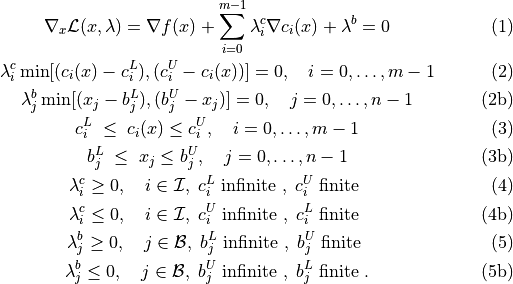Here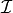and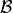represent the sets of indices corresponding to the general inequality constraints and (non-fixed) variable bound constraints respectively. In the conditions above,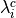is the Lagrange multiplier corresponding to constraint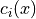, and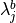is the Lagrange multiplier corresponding to the simple bounds on the variable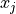. There is exactly one Lagrange multiplier for each constraint and variable. The Lagrange multiplier may be restricted to take on a particular sign depending on whether the corresponding constraint (or variable) is upper bounded or lower bounded, as indicated by (4)-(5). If the constraint (or variable) has both a finite lower and upper bound, then the appropriate sign of the multiplier depends on which bound (if either) is binding (active) at the solution.

In Knitro we define the feasibility error FeasErr at a point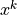to be the maximum violation of the constraints (3), (3b), i.e.,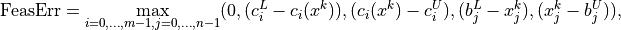while the optimality error (OptErr) is defined as the maximum violation of the first three conditions (1)-(2b), with a small modification to conditions (2) and (2b). In these complementarity conditions, we really only need that either the multiplier or the corresponding constraint is 0, so we change the terms on the left side of these conditions to: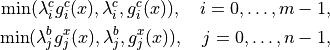where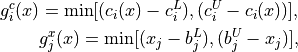to protect against numerical problems that may occur when the Lagrange multipliers become very large. The remaining conditions on the sign of the multipliers (4)-(5b) are enforced explicitly throughout the optimization.

In order to take into account problem scaling in the termination test, the following scaling factors are defined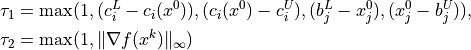where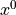represents the initial point.

For unconstrained problems, the scaling factor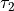is not effective since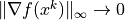as a solution is approached. Therefore, for unconstrained problems only, the following scaling is used in the termination test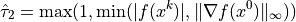in place of.

Knitro stops and declares locally optimal solution found if the following stopping conditions are satisfied: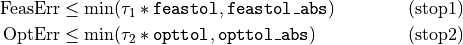where feastol, opttol, feastol_abs, and opttol_abs are constants defined by user options.

This stopping test is designed to give the user much flexibility in deciding when the solution returned by Knitro is accurate enough. By default, Knitro uses a scaled stopping test, while also enforcing that some minimum absolute tolerances for feasibility and optimality are satisfied. One can use a purely absolute stopping test by setting feastol_abs <= feastol and opttol_abs <= opttol.

Note that the optimality condition (1) depends on gradient values of the nonlinear objective and constraint functions. When using finite-difference gradients (e.g. gradopt > 1), there will typically be small errors in the computed gradients that will limit the precision in the solution (and the ability to satisfy the optimality conditions). By default, Knitro will try to estimate these finite-difference gradient errors and terminate when it seems that no more accuracy in the solution is possible. The solution will be treated as optimal as long as it is feasible and the optimality conditions are satisfied either by the optimality tolerances used in (stop2) or the error estimates. This special termination can be disabled by setting findiff_terminate = 0 (none).

Scaling

Note that the optimality conditions (stop2) apply to the problem being solved internally by Knitro. If the user option scale is enabled to perform some scaling of the problem, then the problem objective and constraint functions as well as the variables may first be scaled before the problem is sent to Knitro for the optimization. In this case, the optimality conditions apply to the scaled form of the problem. If the accuracy achieved by Knitro with the default settings is not satisfactory, the user may either decrease the tolerances described above, or try setting scale = no.

Note that scaling the variables or constraints in the problem via the scale user option and scaling/modifying the stopping tolerances are two different things. You should use scale to try to make the variables/constraints in your model all have roughly the same magnitude (e.g. close to 1) so that the Knitro algorithms work better. Separately you should use the Knitro stopping tolerances to specify how much accuracy you require in the solution.

Complementarity constraints

The feasibility error for a complementarity constraint is measured as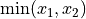where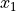and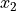are non-negative variables that are complementary to each other. The tolerances defined by (stop1) are used for determining feasibility of complementarity constraints.

Constraint specific feasibility tolerances

By default Knitro applies the same feasibility stopping tolerances feastol / feastol_abs to all constraints. However, it is possible for you to define an (absolute) feasibility tolerance for each individual constraint in case you want to customize how feasible the solution needs to be with respect to each individual constraint.

This can be done using the callable library API functions KN_set_con_feastols(), KN_set_var_feastols(), and KN_set_compcon_feastols(), which allows you to define custom tolerances for the general constraints, the variable bounds and any complementarity constraints. Please see section Callable library API reference for more details on these API functions. When using the AMPL modeling language, the same feature can be used by defining the AMPL input suffixes cfeastol and xfeastol for each constraint or variable in your model.

## Discrete or mixed integer problems

Algorithms for solving optimization problems where one or more of the variables are restricted to take on only discrete values, proceed by solving a sequence of continuous relaxations, where the discrete variables are relaxed such that they can take on any continuous value.

The best global solution of these relaxed problems,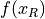, provides a lower bound on the optimal objective value for the original problem (upper bound if maximizing). If a feasible point is found for the relaxed problem that satisfies the discrete restrictions on the variables, then this provides an upper bound on the optimal objective value of the original problem (lower bound if maximizing). We will refer to these feasible points as incumbent points and denote the objective value at an incumbent point by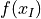. Assuming all the continuous subproblems have been solved to global optimality (if the problem is convex, all local solutions are global solutions), an optimal solution of the original problem is verified when the lower bound and upper bound are equal.

Knitro declares optimality for a discrete problem when the gap between the best (i.e., largest) lower bound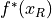and the best (i.e., smallest) upper bound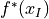is less than a threshold determined by the user options, mip_opt_gap_abs and mip_opt_gap_rel. Specifically, Knitro declares optimality when either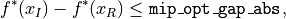or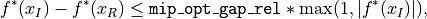where mip_opt_gap_abs and mip_opt_gap_rel are typically small positive numbers.

Since these termination conditions assume that the continuous subproblems are solved to global optimality and Knitro only finds local solutions of non-convex, continuous optimization problems, they are only reliable when solving convex, mixed integer problems. The optimality gap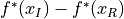should be non-negative although it may become slightly negative from roundoff error, or if the continuous subproblems are not solved to sufficient accuracy. If the optimality gap becomes largely negative, this may be an indication that the model is non-convex, in which case Knitro may not converge to the optimal solution, and will be unable to verify optimality (even if it claims otherwise).